(b)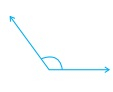(c)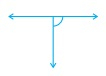(d)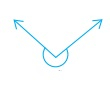(e)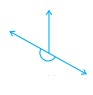(f)" ">

# Classify each one of the following angles as right, straight, acute, obtuse or reflex:(a)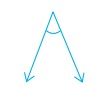(b)(c)(d)(e)(f)"

To do:

We have to classify the given angles as right, straight, acute, obtuse or reflex.

Solution:

(a) The given angle is less than $90^o$.

Therefore, it is an acute angle.

(b) The given angle is more than $90^o$ but less than $180^o$

Therefore, it is an obtuse angle.

(c) The given angle is equal to $90^o$

Therefore, it is a right angle.

(d) The given angle is more than $180^o$ but less than $360^o$

Therefore, it is a reflex angle.

(e) The given angle is equal to $180^o$

Therefore, it is a straight angle.

(f) The given angles are less than $90^o$.

Therefore, they are acute angles.

Updated on: 10-Oct-2022

51 Views